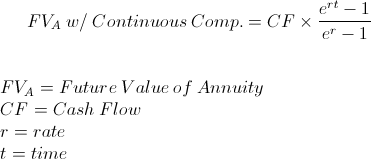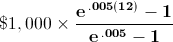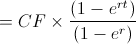# FV of Annuity - Continuous Compounding

FV of Annuity - Continuous Compounding Calculator (Click Here or Scroll Down)The future value (FV) of an annuity with continuous compounding formula is used to calculate the ending balance on a series of periodic payments that are compounded continuously. Understanding the future value of annuity with continuous compounding formula requires the understanding of two specific financial and mathematical concepts, which are future value of an annuity and continuous compounding.

## Future Value of Annuity, Continuous Compounding, and Geometric Series

Future Value of Annuity - The future value of an annuity is the sum of a series of periodic payments and typically involves compounding of interest as the balance increases. The formula for future value of annuity alone generally solves the question "How much will I have saved at X dollars per month after Y months."

Continuous Compounding - Continuous compounding is compounding that is constant. One way some try to visualize the concept of continuous compounding is that is fluid, constantly compounding moment by moment, as opposed to daily, monthly, quarterly, or annually. The question a few sentences above regarding 'How much will I have saved' must also take into consideration how often interest is compounded in the interest bearing account. The formula for continuous compounding isThe future value of annuity with continuous compounding formula applies both of these concepts for one saving in an account that has continuous compounding.

## Example of the FV of Annuity with Continuous Compounding Formula

Using the example in the preceding section, assume an individual is wanting to determine the ending balance after 1 year based on monthly deposits of \$1,000 in an account that has 6% continuous compounding.

*It is important to keep in mind that the initial deposit will be at period 1 and not immediately. Broadly speaking, an initial cash flow (deposit, in this case) would apply to 'annuity due' and not a simple annuity.*

Applying the future value of annuity with continuous compounding to this example would showwhich would return a result of \$12,336.42. Note that the rate used is .005, or
.5%, which is the monthly rate for a 6% annual rate. This can be checked with the calculator on the bottom of the page.

It may seem as if compounding nonstop will produce far more than monthly compounding, but for the sake of comparison, the same cash flows at the same rate would have an ending balance of \$12,335.56.

*Please keep in mind that this is the mathematical concept but real results may vary due to rounding, time of deposit, and for various other reasons.

## How is the Future Value of Annuity with Continuous Compounding Derived?

The future value of annuity with continuous compounding formula is the sum of future cash flows with interest. The sum of cash flows with continuous compounding can be shown asThis is considered a geometric series as the cash flows are all equal. The common ratio for this example is er. The geometric series formula for the formula above showsThe entire formula above can be multiplied by -1/-1 to get the formula at the top of the page.

New to Finance?